Related Tags

euler
algorithm
communitycreator

# Project Euler 33: digit canceling fractions

Armaan Nougai

### Problem statement

We have a fraction in which if we cancel the common digit from the numerator and denominator, the fraction thus obtained is an equivalent fraction. This type of fraction is called a curious fraction.

There are indeed only four curious fractions whose actual value is less than one, with two digits in the numerator and denominator.

ex . 49/98 = 4/8

### Find the denominator of the product of such fractions when expressed in the lowest terms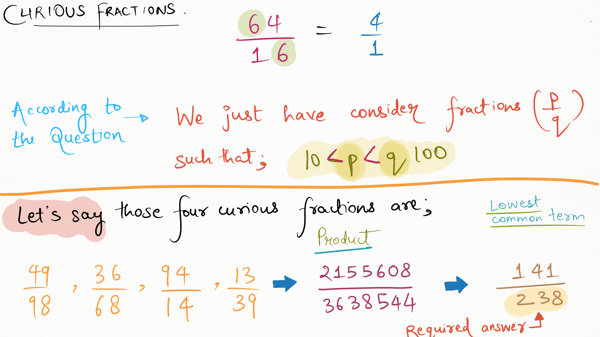Question analysis

### Solution

Suppose the following:

p/q = original fraction
i = digit to be cancelled
n/d = remaining fraction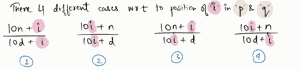### Analyzing cases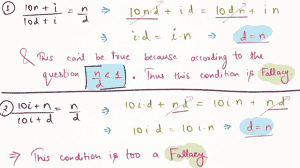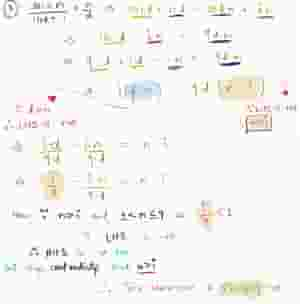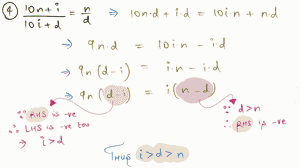After analyzing, we conclude that:

1. Out of four possible conditions, only the fourth condition will be true for a curious fraction less than one.

2. i > d > n

### Code

def gcd(a,b):
b, a= min((a, b)), max((a, b))
if a%b == 0: return b

# if any of a or b is odd, then we will skip all odd factors
any_odd = (a|b)%2
hcf = 1
for j in range(2 + any_odd, b//2 + 1, 1 + any_odd):
print('checking',j)
if b%j == 0 and a%j == 0:
hcf = j
return hcf

# num/den is the multiplication of all curious fraction less than 1
num = 1
den = 1

# i is digit to be cancelled
for i in range(1, 10):
# d is remaining digit in denominator
for d in range(1, i):
# n is remaining digit in numerator
for n in range(1, d):
if 10*n*d + i*d == 10*i*n + d*n:
num *= 10*n + i
den *= 10*i + d

common_term = gcd(num, den)

print(den//common_term)

RELATED TAGS

euler
algorithm
communitycreator

CONTRIBUTOR

Armaan Nougai
RELATED COURSES

View all Courses

Keep Exploring

Learn in-demand tech skills in half the time